Question

# Write the net ionic equation for the reaction that occurs when equal volumes of 0.205 M...

Write the net ionic equation for the reaction that occurs when equal volumes of 0.205 M aqueous hypochlorous acid and ethylamine are mixed. It is not necessary to include states such as (aq) or (s).
At equilibrium the (reactants OR products) will be favored.

The pH of the resulting solution will be (greater than /equal to/ or less than) seven.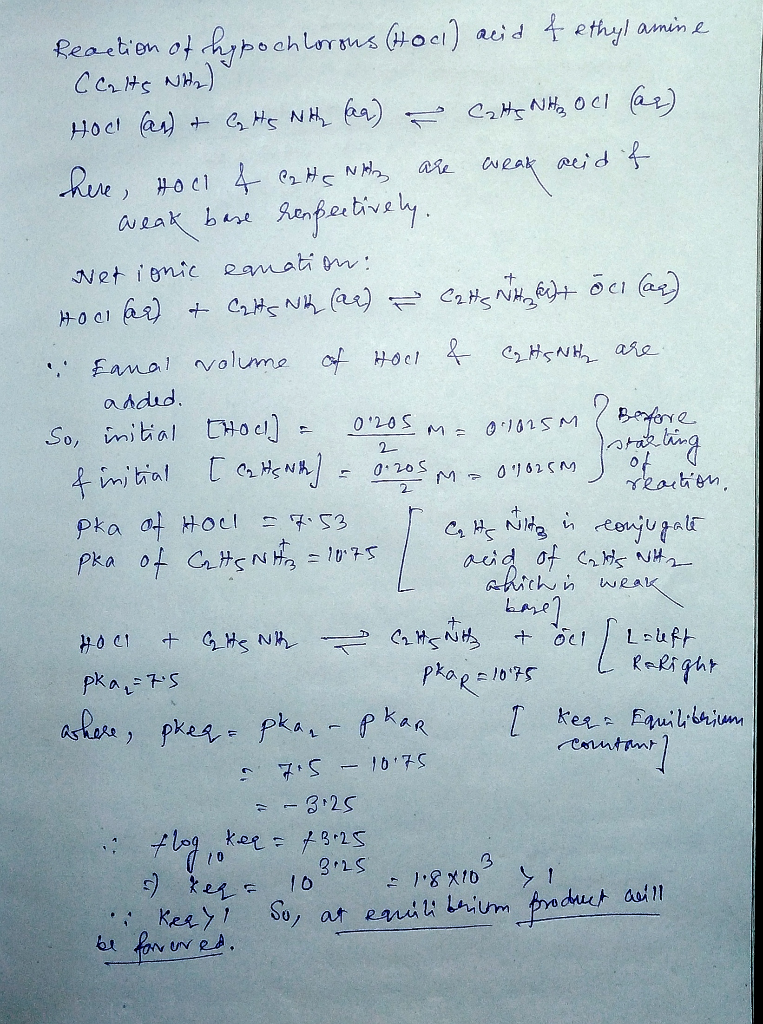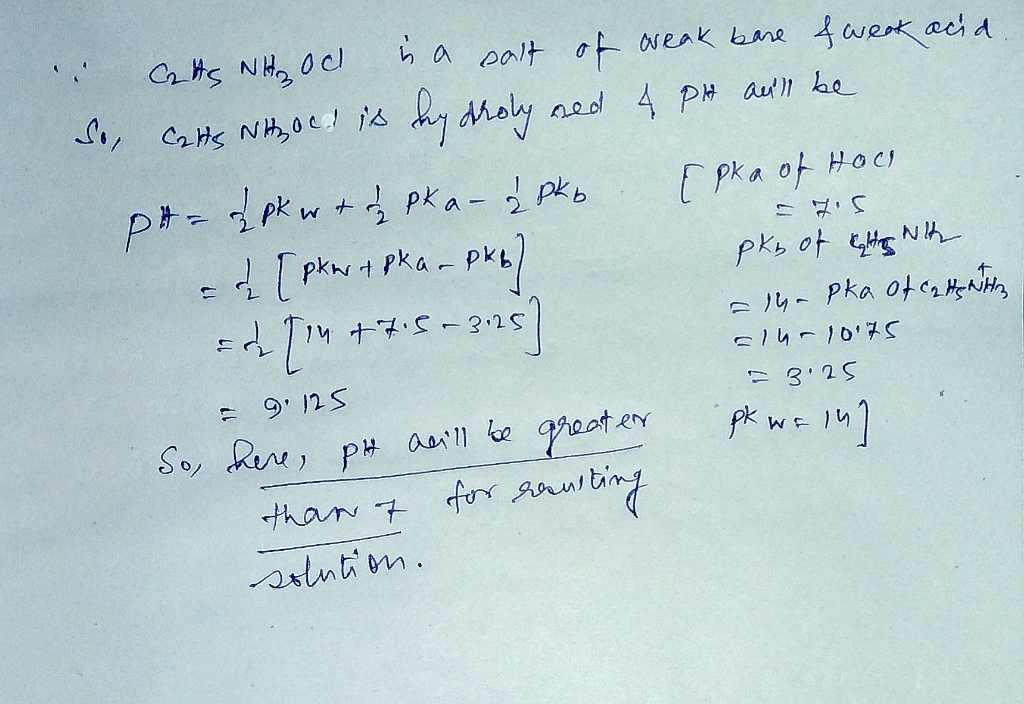#### Earn Coins

Coins can be redeemed for fabulous gifts.

Similar Homework Help Questions
• ### (1) Given the following information: hydrocyanic acid HCN Ka = 4.0×10-10 diethylamine (C2H5)2NH Kb = 6.9×10-4...

(1) Given the following information: hydrocyanic acid HCN Ka = 4.0×10-10 diethylamine (C2H5)2NH Kb = 6.9×10-4 (1) Write the net ionic equation for the reaction that occurs when equal volumes of 0.152 M aqueous hydrocyanic acid and diethylamine are mixed. It is not necessary to include states such as (aq) or (s). (2) At equilibrium will the reactants or products be favored? (3) Will the pH of the resulting solution be greater than, less than, or roughly equal to 7?...

• ### Given the following information: hypochlorous acid HCIO CoH3COOH Ka = 3.5x10-8 Kg = 6.3x10-5 benzoic acid...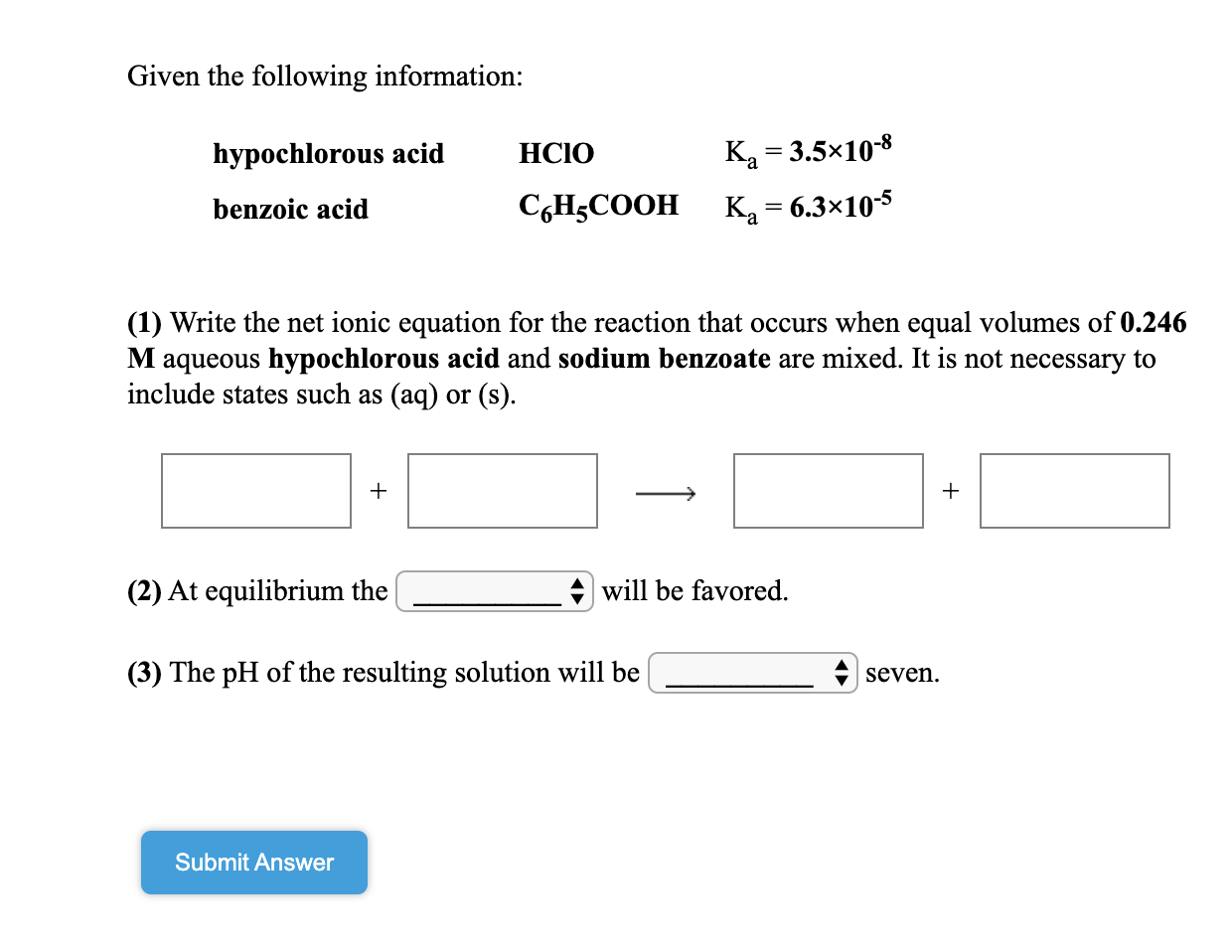Given the following information: hypochlorous acid HCIO CoH3COOH Ka = 3.5x10-8 Kg = 6.3x10-5 benzoic acid (1) Write the net ionic equation for the reaction that occurs when equal volumes of 0.246 M aqueous hypochlorous acid and sodium benzoate are mixed. It is not necessary to include states such as (aq) or (s). (2) At equilibrium the will be favored. (3) The pH of the resulting solution will be _ seven. Submit Answer

• ### Given the following information: HNO nitrous acid ammonia K, -4.5x10+ Kb = 1.8x105 NH3 (1) Write...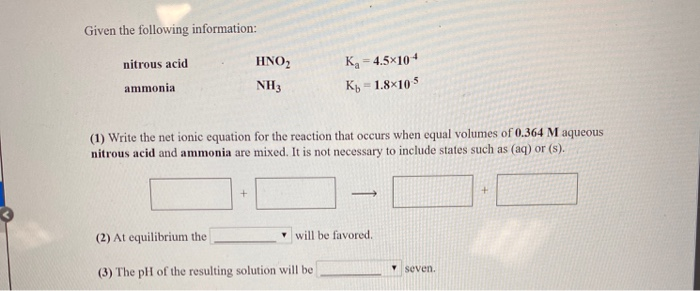Given the following information: HNO nitrous acid ammonia K, -4.5x10+ Kb = 1.8x105 NH3 (1) Write the net ionic equation for the reaction that occurs when equal volumes of 0.364 M aqueous nitrous acid and ammonia are mixed. It is not necessary to include states such as (aq) or (s). (2) At equilibrium the will be favored (3) The pH of the resulting solution will be seven.

• ### Given the following information: K, = 3.0x10-4 acetylsalicylic acid (aspirin) triethylamine (C2H3)3N Kb = 5.2*10-4 (1)...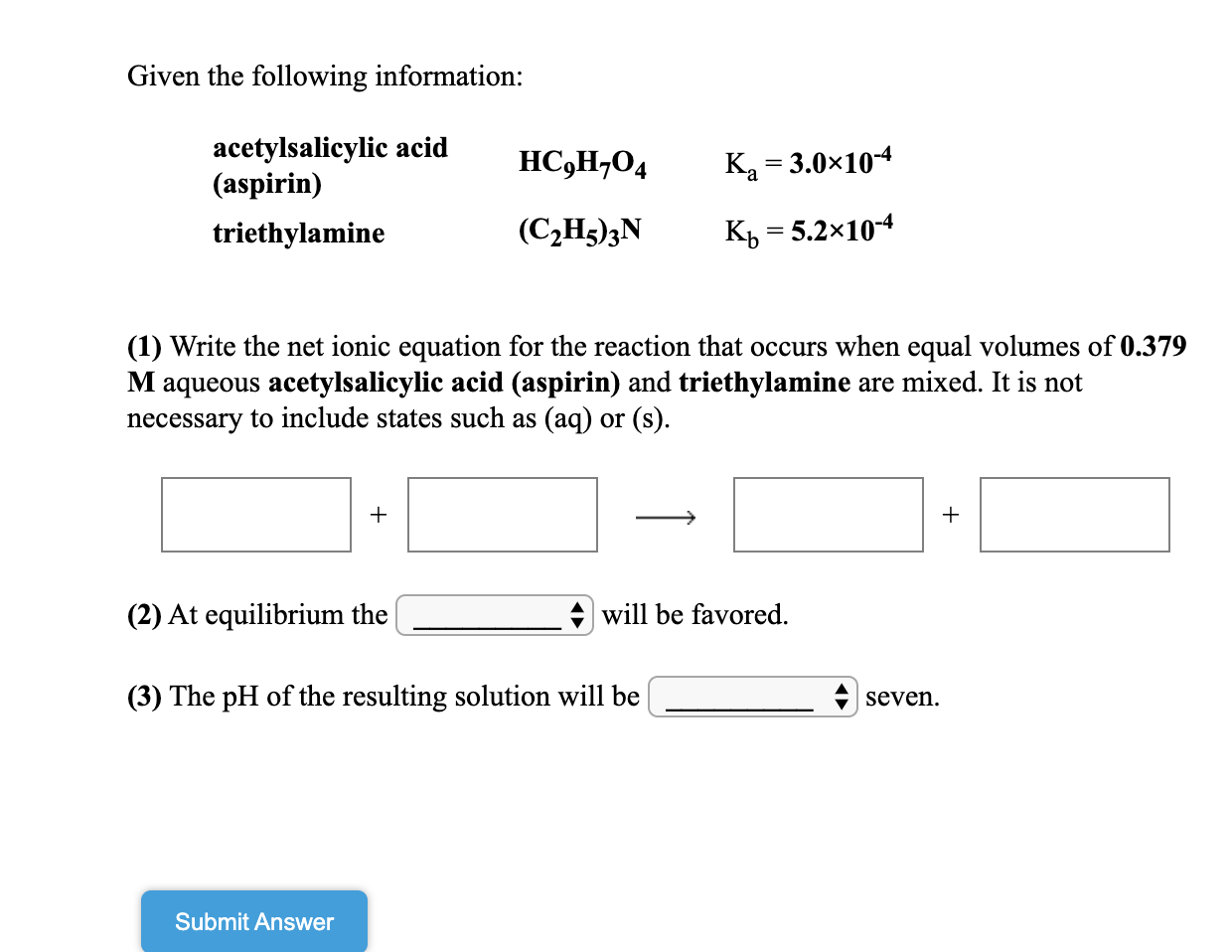Given the following information: K, = 3.0x10-4 acetylsalicylic acid (aspirin) triethylamine (C2H3)3N Kb = 5.2*10-4 (1) Write the net ionic equation for the reaction that occurs when equal volumes of 0.379 M aqueous acetylsalicylic acid (aspirin) and triethylamine are mixed. It is not necessary to include states such as (aq) or (s). (2) At equilibrium the will be favored. (3) The pH of the resulting solution will be _ A seven. Submit Answer

• ### Given the following information: - HF hydrofluoric acid hypochlorous acid HCIO HF is a stronger acid...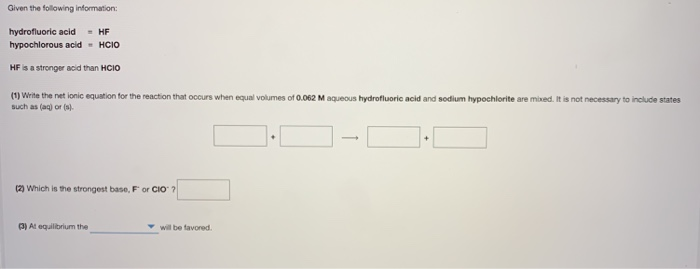Given the following information: - HF hydrofluoric acid hypochlorous acid HCIO HF is a stronger acid than HCIO (1) Write the net ionic equation for the reaction that occurs when equal volumes of 0.062M aqueous hydrofluoric acid and sodium hypochlorite are mixed. It is not necessary to include states such as (aq) or (s) (2) Which is the strongest base, F or CIO ) At equilibrium the will be favored

• ### Given the following information: hydrofluoric acid HF acetic acidCH3COOH HF is a stronger acid than CH3COOH...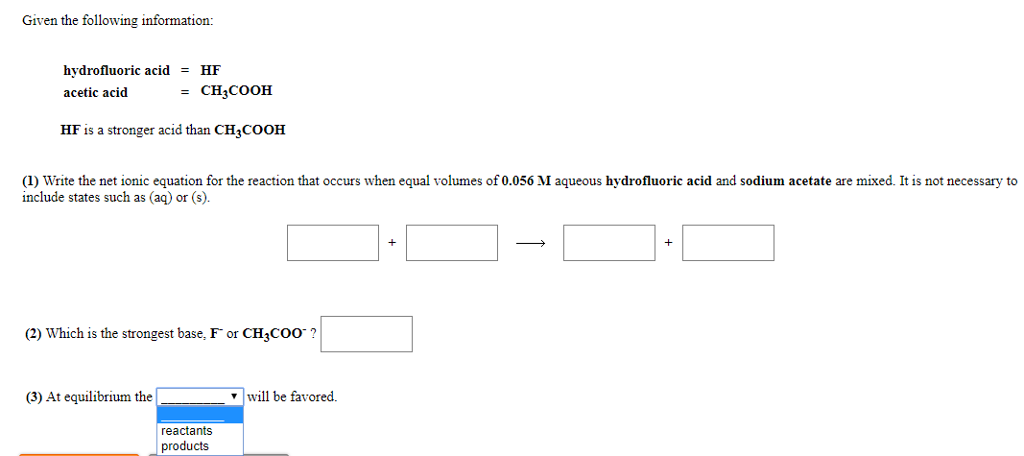Given the following information: hydrofluoric acid HF acetic acidCH3COOH HF is a stronger acid than CH3COOH (1) Write the net ionic equation for the reaction that occurs when equal volumes of 0.056 M aqueous hydrofluoric acid and sodium acetate are mixed. It is not necessary to include states such as (aq) or (s). (2) Which is the strongest base, F or CH3coo? (3) At equilibrium the 7 will be favored. reactants products

• ### Equal volumes of 0.298 M aqueous solutions of acetic acid (CH3COOH(aq)) and sodium formate (NaHCO...

Equal volumes of 0.298 M aqueous solutions of acetic acid (CH3COOH(aq)) and sodium formate (NaHCOO) are mixed. (1) Write the net ionic equation for the overall reaction that takes place as the system comes to equilibrium. Write acetic acid, benzoic acid or formic acid and their conjugates in the form RCOOH / RCOO-. For example, benzoic acid should be written "C6H5COOH" NOT "C6H5CO2H". It is not necessary to include states such as (aq). (2) What is the value of the...

• ### Dropdown is products or reactants. Given the following information: = formic acid hydrocyanic acid HCOOH HCN...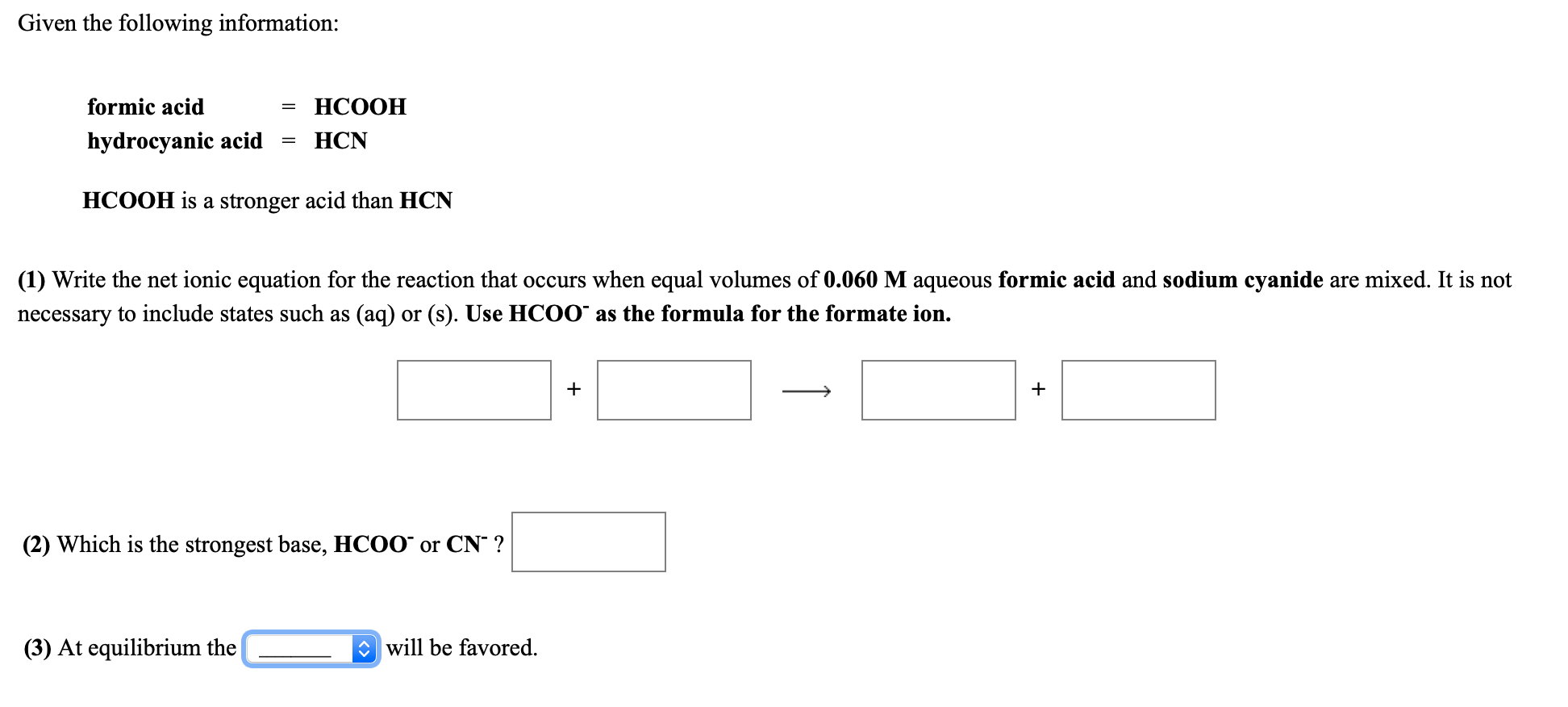Dropdown is products or reactants. Given the following information: = formic acid hydrocyanic acid HCOOH HCN HCOOH is a stronger acid than HCN (1) Write the net ionic equation for the reaction that occurs when equal volumes of 0.060 M aqueous formic acid and sodium cyanide are mixed. It is not necessary to include states such as (aq) or (s). Use HCOO as the formula for the formate ion. + + (2) Which is the strongest base, HCOO or CNº...

• ### I need help on this question. Thank you Equal volumes of 0.248 M aqueous solutions of...

I need help on this question. Thank you Equal volumes of 0.248 M aqueous solutions of nitrous acid (HNO2(aq)) and sodium benzoate (NaC6H5COO) are mixed. (1) Write the net ionic equation for the overall reaction that takes place as the system comes to equilibrium. Write acetic acid, benzoic acid or formic acid and their conjugates in the form RCOOH / RCOO-. For example, benzoic acid should be written "C6H5COOH" NOT "C6H5CO2H". It is not necessary to include states such as...

• ### a.Write the balanced NET IONIC equation for the reaction that occurs when perchloric acid and sodium...a.Write the balanced NET IONIC equation for the reaction that occurs when perchloric acid and sodium hypochlorite are combined. It is not necessary to include states such as (aq) or (s). + + This reaction is classified as:  _________Strong Acid + Strong BaseWeak Acid + Strong BaseStrong Acid + Weak BaseWeak Acid + Weak Base. The extent of this reaction is: _________Below 50%50%Above 50%100%. When 25.0 mL of 0.300 M perchloric acid and 25.0 mL of 0.300 M sodium hypochlorite are...